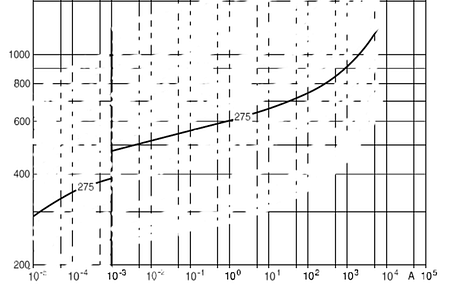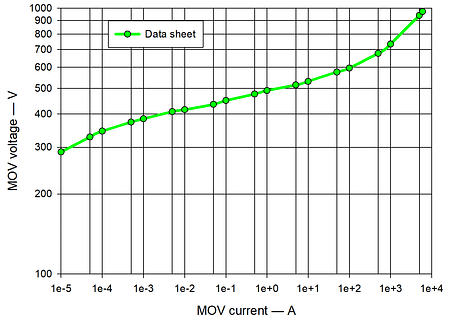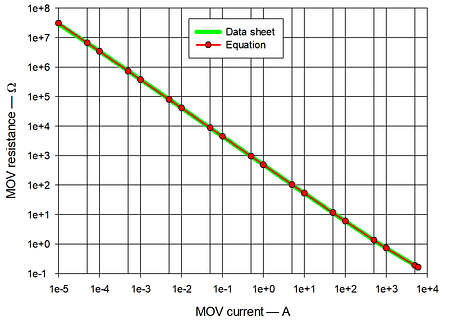# 275 V 14 mm MOV characteristic modelling over 9 current decades

The previous two contributions showed there is a strong correlation between metal-oxide varistor (MOV) current and resistance. This means there is also a strong correlation between MOV current and voltage making an MOV a current dependent resistor rather than being a voltage dependent resistor. This misconception probably came about as we are used to applying a variable voltage to components to measure their  VI characteristic rather than a variable current. Previously, a high current MOV example was used, which gave a relationship of V = 815 + 4.32*(I)0.51 over a current range of 300 A to 50 kA. Predominately, the voltage varies as the square root of the current and the resistance varies as the reciprocal of the square root of the current. The steps in analyzing the 14 mm 275 V MOV example here are; log the data sheet VI characteristic values, unify those values, curve fit the resultant values to produce the characteristic equation valid over an extended current range of 10 µA to 6 kA.
The starting point was the published data sheet VI curve for the 275 V member of  a 14 mm MOV family. For clarity, the curves for the other voltage members of the MOV family have been (white) redacted, see Figure 1.Figure 1 – 275 V 14 mm MOV data sheet VI curve

The Figure 1 values at specific currents were measured in a 1, 5 sequence and recorded. Accurately measuring values on no-linear logarithmic scales is difficult. By having a logarithmic reference scale held at an angle to match up with the graph decade lines, intermediate values are easier to determine. Logarithmic reference scales such as printed logarithmic graph paper or, as in this case, the slider of a slide rule can be used.  Figure 1 shows a step in the characteristic at 10-3 A as the characteristic moves from typical voltage to maximum voltage. This step needs to be removed by normalizing the maximum voltage values down to the typical curve by reducing the maximum value curve above 10-3 A down the match the typical curve below 10-3 A, giving the Figure 2 data plot.Figure 2 – Transcribed 275 V 14 mm MOV VI values

Using the resultant V and I values of Figure 2, the resistance, R (=V/I) was calculated (green line) and the most suitable resistance versus current equation found (red line), see Figure 3.Figure 3 – Resistance versus current plot for 275 V 14 mm MOV

Figure 3 shows the strong logarithmic correlation between MOV current and resistance. In preparing data for curve-fitting to various equations it is logical to pre-process wide range data into LOG form if the final result of the current equation is to be expressed on a logarithmic axes. Further the logarithmic data is likely to have a smaller range than the original data, which helps balance the fit at high and low values. As an extreme range case, like resistance, one could offset the logarithmic resistance data for equal positive and negative values, making the highest and lowest data values have equal errors in the curve fit. Figure 4 shows an equation curve-fitting result where the LOG(R) range has been normalized to have equal peak values of ±4.125 as shown in Figure 4.Figure 4 – Normalized logarithmic Resistance values versus current and 3-term power law equation constant values

The resultant equation for typical voltage versus current gave V = 482 + 4.59*(I)0.5 + 15.4*LN(I). The first two parts of the equation amount to a 3-term power law. The third part, 15.4*LN(I) is interesting as it develops a negative voltage when the current falls below 1 A. This negative voltage subtracts from the 3-term power law fixed voltage of 482 V. Example, 15.4*LN(I)  values at 1 A, 0.01 A and 0.0001 A are 0 V, -71 V and -142 V. At 0.00001 A  the equation voltage is 305 V.   Figure 4 plots this equation (red line) over the starting normalized data (green line)  and, again, the voltage predominantly varies as the square root of the current.

The maximum voltage curve equation will be V = 595.5 + 5.67*(I)0.5 + 19.03*LN(I).